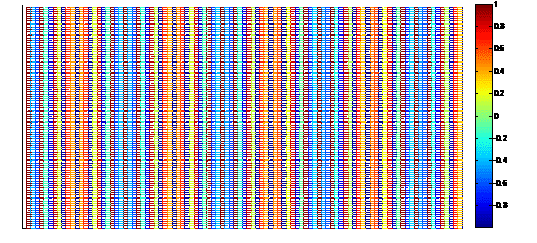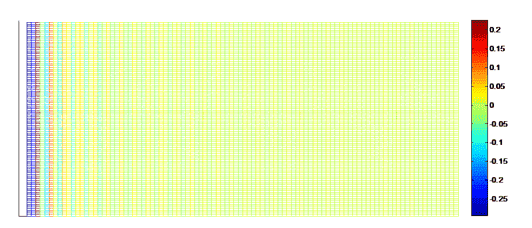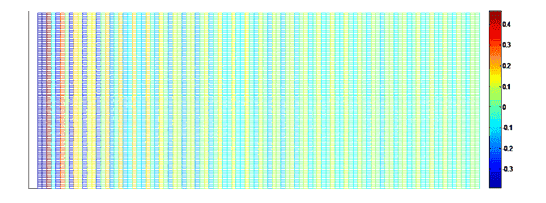# Need 2D plot of plane wave, cylindrical & spherical wave

plane wave is represented as exp (ik.r) and the
cylindrical wave as 1/sqrt(r) *exp(ik.r) and the
spherical wave as 1/r*exp(ik.r)

Have any one tried to plot these waves? How to do it?

Attempt: in Matlab assuming k=1

>> x=linspace(-1,1,100);
>> for(ii=1:100)
fp(ii)=exp(i*x(ii));
end
>> [x,fp]=meshgrid(x,fp);
>> mesh(x,real(fp))

gave me plane surface that is inclined in the graph however I still need to create Cylindrical wave and spherical wave. I am confused on how to use " r " for cylindrical and spherical wavefronts.

BvU
Homework Helper
Hello Allam, welcome to PF :)

I understand the planar wave expression, ##{\bf k}## and ##{\bf r}## are vectors and the inner product ##{\bf k}\cdot{\bf r}## makes it a planar wave.
What you then render seems one-dimensional to me (not familiar with matlab :( ), k has disappeared (probably ok: k = 1,0,0 or so).

##i## in Matlab ? is ##i^2 = -1## ?

Is 1/sqrt(r) *exp(ik.r) really the expression for a cylindrical wave (yes, Bessel function for large ##|{\bf r}|## -- you confused me because I read it as ##{\bf k}\cdot{\bf r}## again, but you mean ## kr##)
You could render this similarly one-dimensionally along a ray. Same for the spherical guy.

I suppose you did google planar wave images and cylindrical wave images already ? All struggle with the problem that you need too many dimensions if you want a full picture.

I assumed k=1 and ignored the time dependence.
By the By k is not vector it is scalar and it is exp(ik(n.r)) where n is unit normal vector to r

Iam interested in the variation of amplitude in the space. I have developed Matlab code for plotting and in the plot from the color code one can see that amplitudevaries differently for different waves.

BvU
Homework Helper
Strange. Amplitude for planar wave should be a constant.
Looking at the matlab code, I suppose the plot shows the real part only.

And if ## {\bf n} \perp {\bf r} ##, then ##{\bf n} \cdot {\bf r} = 0##.

I wonder what I see in the pictures. Top one shows about 4.5 periods of a plane wave in the horizontal direction. Vertically ?
Lower two pics ?

True , Thank You for correcting me in that n is parallel to r. n is unit vector in the direction of propagation and r is the position vector. I have written another matlab code which goes like this for plane wave generation:
x=linspace(0,99);
y=linspace(0,99);
r=sqrt((x.*x)+(y.*y));
for j=1:100
pw(j)=exp(i*(x(j)+y(j)));
end
[r,pw]=meshgrid(r,pw);
mesh(real(pw))
All the 3 pictures show propagation horizontally. In the first one as you rightly said there are 4.5 periods and beam does not diverge.it carries forward its intensity which is constant. In the second and the third the spherical and the cylindrical diverge and intensity diminishes rapidly in case of spherical than cylindrical. the plot actually gives the intensity information and not amplitude.since intensity is square(amplitude). it goes in line. Yeah!! In matlab "i" means imaginary number of value sqrt(-1).

please be informed its not the intensity!!! i guess it is just the real part of complex amplitude !!

BvU